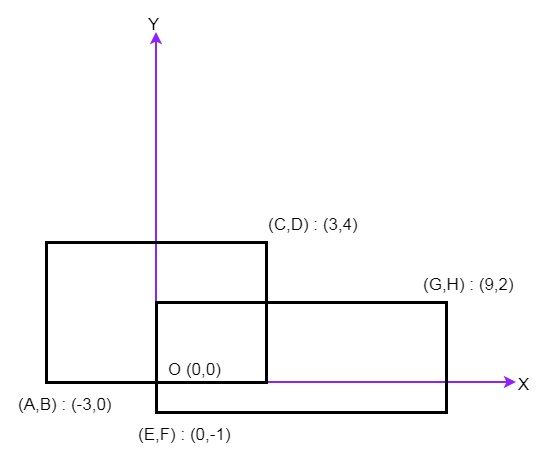# Rectangle Area in C++

Suppose we want to find the total area covered by two rectilinear rectangles in a 2D plane. Here each rectangle is defined by its bottom left corner and top right corner as shown in the figure.To solve this, we will follow these steps −

• if C = E or A >= G or B >= H or D <= F, then

• return (C – A) * (D – B) + (G – E) * (H – F)

• Define an array h, insert A, C, E, G into h

• Define an array v, insert B, D, F, H into v

• sort h array and sort v array

• temp := (h – h) * (v – v)

• total := temp

• total := total + (C – A) * (D – B)

• total := total + (G – E) * (H – F)

## Example(C++)

Let us see the following implementation to get better understanding −

Live Demo

#include <bits/stdc++.h>
using namespace std;
class Solution {
public:
int computeArea(int A, int B, int C, int D, int E, int F, int G, int H) {
if(C <= E || A >= G || B >= H || D <= F) return (C - A) * (D - B) + (G - E) * (H - F);
vector <int> h;
h.push_back(A);
h.push_back(C);
h.push_back(E);
h.push_back(G);
vector <int> v;
v.push_back(B);
v.push_back(D);
v.push_back(F);
v.push_back(H);
sort(h.begin(), h.end());
sort(v.begin(), v.end());
long long int temp = (h - h) * (v - v);
long long int total = - temp;
total += (C - A) * (D - B);
total += (G - E) * (H - F);
}
};
main(){
Solution ob;
cout << (ob.computeArea(-3, 0, 3, 4, 0, -1, 9, 2));
}

## Input

-3
0
3
4
0
-1
9
2

## Output

45